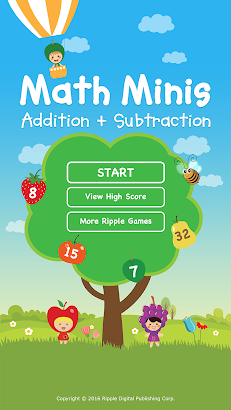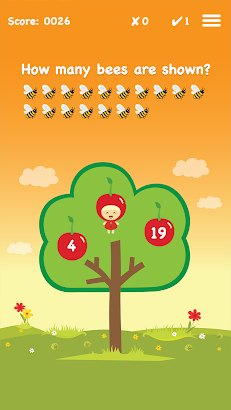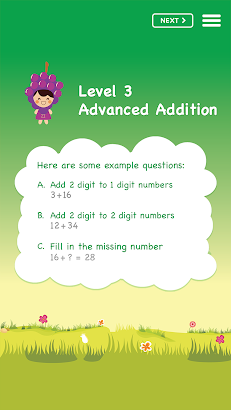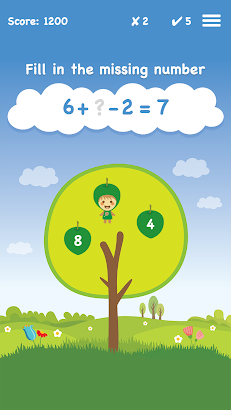# Math Minis

## More fun than a math workbook – learn to add and subtract in 8 different levels.

User Rating: ( 3 ratings )

### Screenshot Math Minis### See Description

Math Minis – Addition and Subtraction.

More fun than a math workbook – select the correct answer and the fruit comes alive with cute animation. Features 8 different levels of adding and subtracting skill sets. Each level has a different background, tree and fruits that turn into little people. Perfect for preparing preschooler into their first year of elementary school. This app have built in instructional voice over and user can select any level to play.

Level 1 – Counting and Number Patterns
• Counting Review
• Number Sequence
• Number Patterns

• Add 3 single digit numbers

• Add 2 digits to 1 digit numbers
• Add 2 digits to 2 digit numbers
• Fill in the missing number

Level 4 – Basic Subtraction
• Subtract single digit numbers
• Subtract 3 single digit numbers
• Subtracting 10s

• Subtract 1 digit from 2 digit numbers
• Subtract 2 digits from 2 digit numbers
• Fill in the missing number

Level 6 – Addition and Subtraction Review
• Mixed questions of addition and subtraction with single digit numbers
• Mixed questions of addition and subtraction with 3 single digit numbers
• Mixed questions of adding and subtracting 10s

Level 7 – Combining Addition and Subtraction
• Add and subtract single digit number
• Add and subtract 1 and 2 digit numbers
• Fill in the missing number

Level 8 – Final Challenge
• Add 2 digits to 2 digit numbers
• Subtract 2 digits from 3 digit numbers
• Fill in the missing number

The first 4 levels are free. There is a level of parental security to prevent children from accessing features outside of this app including making in-app purchases.

If you like this app, visit http://www.ripplepublishing.ca for more fun apps

 App Name Math Minis Developer Ripple Digital Publishing Requirements Android 2.3.3 and up Package ca.ripplepublishing.mathminis
Tags
Close